Learning Library
Who we are
Guidance

# 3.NF.A Worksheets, Workbooks, Lesson Plans, and Games

#### CCSS.MATH.CONTENT.3.NF.A

:
"Develop understanding of fractions as numbers."

These worksheets and exercises can help students practice this Common Core State Standards skill.

## Worksheets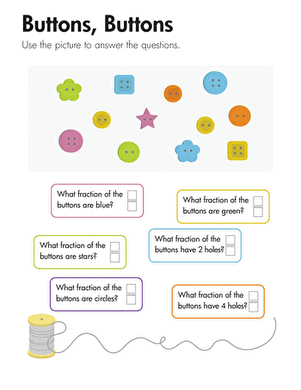Fractions: Buttons, Buttons
Worksheet
Fractions: Buttons, Buttons
This third grade math worksheet asks questions about a set of buttons. Kids respond to the questions by counting to determine the fractions.
Math
Worksheet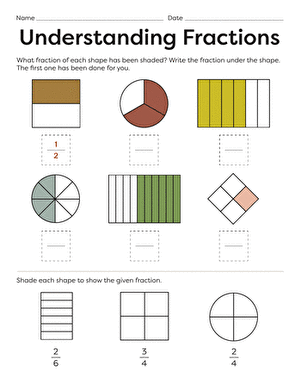Fun Fractions
Worksheet
Fun Fractions
Each shape on this third grade math worksheet is divided into equal parts, and some of the parts are shaded. Kids identify and write the fraction represented.
Math
Worksheet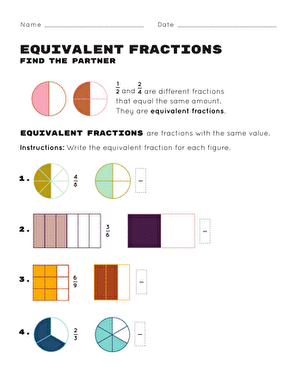Equivalent Fractions: Find the Partner
Worksheet
Equivalent Fractions: Find the Partner
This equivalent fractions worksheet helps your child learn about and create equivalent fractions. Try this equivalent fractions worksheet with your child.
Math
Worksheet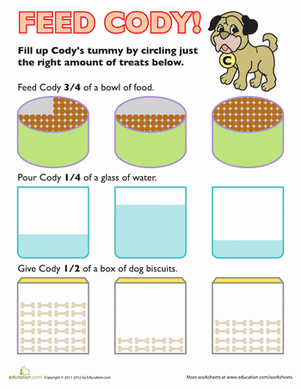Fraction Pictures
Worksheet
Fraction Pictures
To feed Cody, your child will have to decide which fraction picture fits the number fraction given: 3/4, 1/4 or 1/2.
Math
Worksheet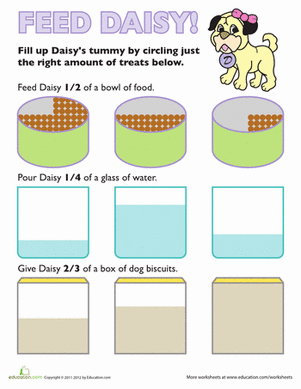Food Fractions: Feed Daisy
Worksheet
Food Fractions: Feed Daisy
With this visual fraction sheet, your child will have to determine which fraction picture matches the fraction number given in order to feed Daisy.
Math
WorksheetFood Fractions: Feed Fido
Worksheet
Food Fractions: Feed Fido
Can your child help feed Fido? He'll decide which fraction picture matches the fraction number given.
Math
Worksheet

## Lesson Plans

No plans found for this common core node.

## Workbooks

No workbooks found for this common core node.

## Games

No games found for this common core node.

## Exercises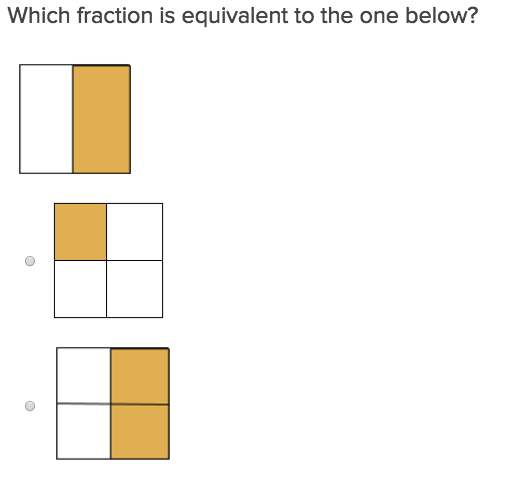Equivalent Fractions 1
Exercise
Equivalent Fractions 1
Equivalent Fractions will help students practice this key third grade skill. Try our free exercises to build knowledge and confidence.
Math
Exercise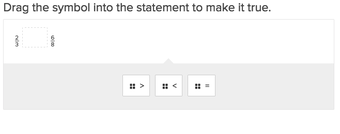Compare Fractions with Different Denominators 1
Exercise
Compare Fractions with Different Denominators 1
This exercise will explain to students the confusing task of comparing fractions with different denominators.
Math
Exercise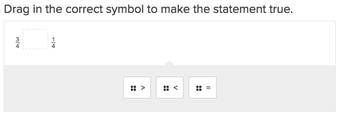Compare Fractions with the Same Denominators 1
Exercise
Compare Fractions with the Same Denominators 1
Students will love comparing fractions with the same denominators once they work through this exercise.